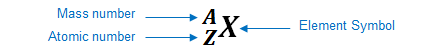# Problem: What species is represented by the following information?p+ = 47            n° = 62                       e- = 46A) Ag+     B) Pd+     C) Ag     D) Nd     E) Pd

###### FREE Expert Solution

We’re being asked to determine the species that is represented by the given subatomic particles.

An element can be represented as:You can determine the identity of the element using its atomic number:

Atomic number (Z):

represents the identity of the element
reveals the number of protons
in a neutral element:

# of protons = # of electrons

Mass number (A)

reveals the number of protons and neutrons

mass number = # of protons + # of neutrons

Given:###### Problem Details

What species is represented by the following information?

p+ = 47            n° = 62                       e- = 46

A) Ag+

B) Pd+

C) Ag

D) Nd

E) Pd

What scientific concept do you need to know in order to solve this problem?

Our tutors have indicated that to solve this problem you will need to apply the Subatomic Particles concept. You can view video lessons to learn Subatomic Particles. Or if you need more Subatomic Particles practice, you can also practice Subatomic Particles practice problems.

What is the difficulty of this problem?

Our tutors rated the difficulty ofWhat species is represented by the following information?p+ ...as low difficulty.

How long does this problem take to solve?

Our expert Chemistry tutor, Sabrina took 3 minutes and 9 seconds to solve this problem. You can follow their steps in the video explanation above.

What professor is this problem relevant for?

Based on our data, we think this problem is relevant for Professor Carpenter & Hamilton's class at UMBC.